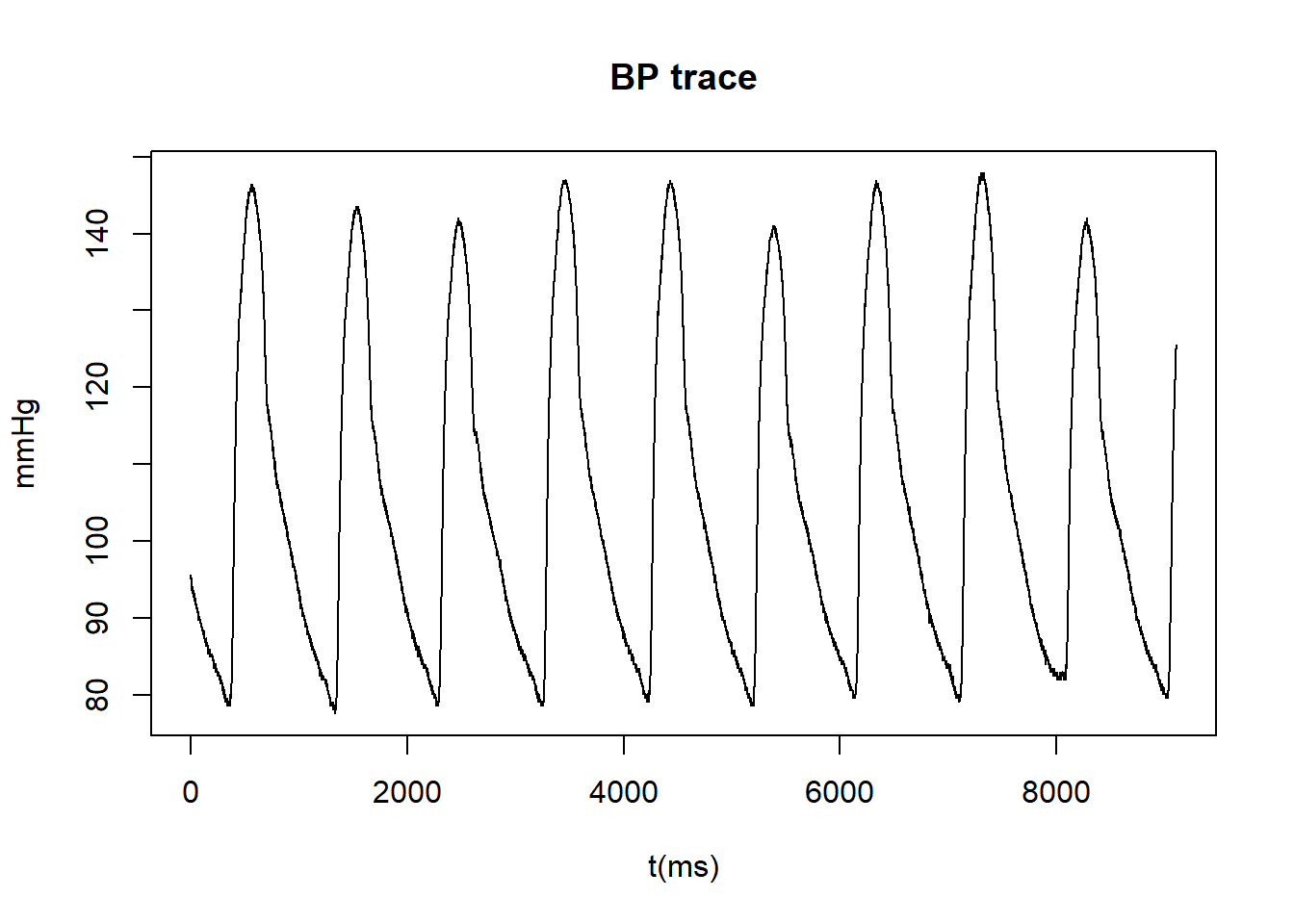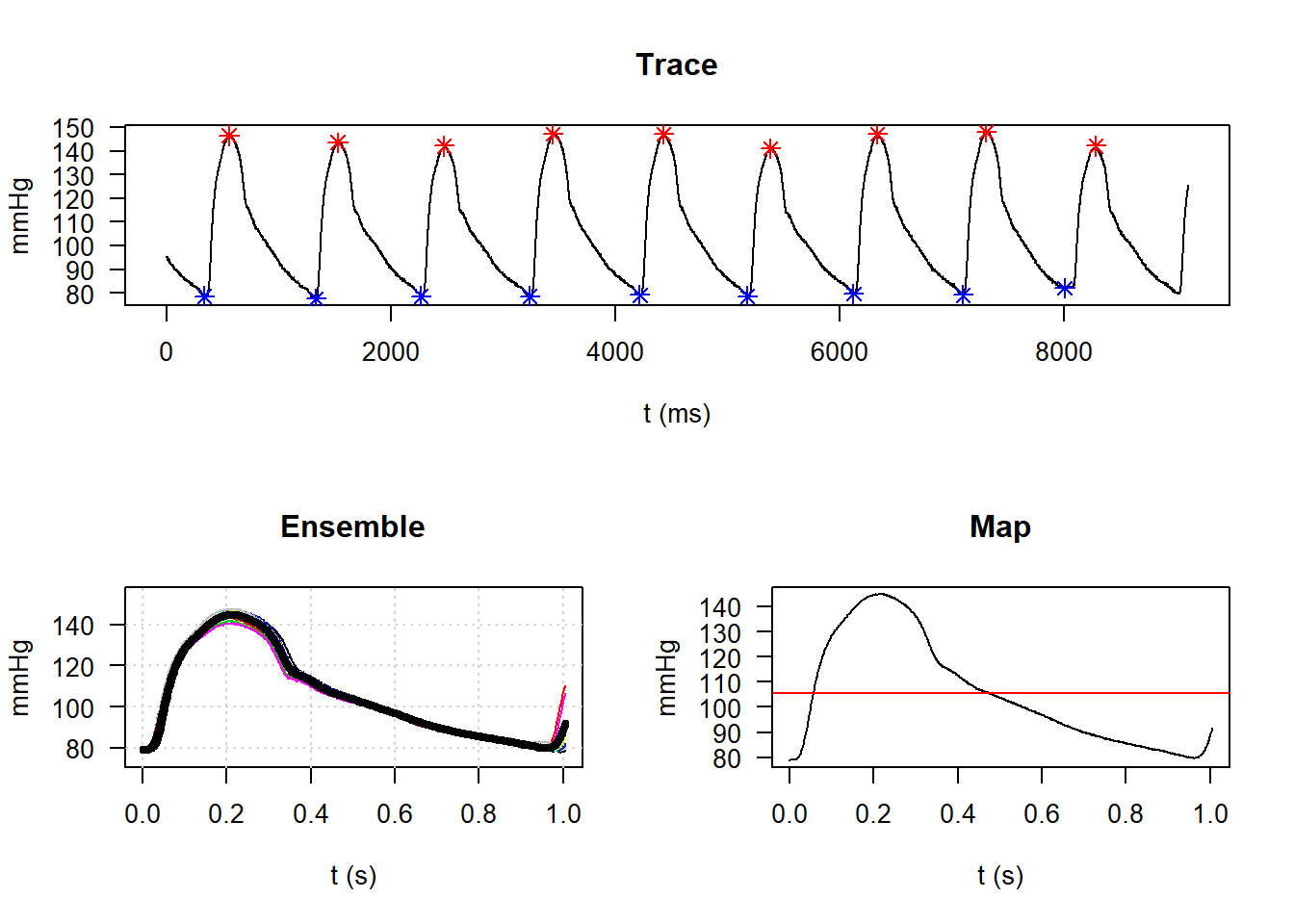## R: Ensemble averaged blood pressure waveforms

As part of my research I deal with blood pressure (BP) waveforms. Often it is useful to ensemble average a continous trace of BP waveforms (spanning multiple cardiac cycles) into a single ensemble average beat (spanning one cardiac cycle). Currently we do this using Matlab, but I have been working on moving this functionality into R in my spare time. This post will detail where I am at.

First, we load the blood pressure trace. This is a single file (txt or csv) with a single column which contains the data.

BP = read.table("C:/Users/mka4/Desktop/pressure.txt", dec=".") #load text file

To view the data we could simply plot it out. Always good to check!

p = BP$V1 plot(p, type ="l", ylab = "mmHg", xlab = "t(ms)", main = "BP trace")Next comes the trickier part. We need to detect individual cardiac cycles and average the values for each beat to give a single ensembled averaged beat. Below is my function for doing this. Cardiac cycles are detected using the nadir (diastole) of the waveform trace. ensemble = function(P){ P = P$V1                    # loading data
ns = 1000                   # sample rate
nd = 250                    # lag factor to pic beats

# Find troughs -----------------------------------------------------------
a = min(p[1:ns])            # find 1st trough
nmin = which(p[1:ns]==a)    # time @ first trough
nmin = nmin
j = 1                       # nmax= index corresponding to maximum peak
nb = NULL
nb[j] = nmin

# find all troughs
nend = nb[j] + nd + ns
suppressWarnings ( while (nb[j] + nd + ns < length(p)) {
nstart = nb[j] + nd
nend = nstart + ns
a = min(p[nstart:nend])
nmin = which(p[nstart:nend] == a)
j = j + 1
nb[j] = nmin + nstart - 1} )

# Find peaks ---------------------------------------------------------------
v = max(p[1:ns])            # find 1st peak
nmax = which(p[1:ns]==v)    # time @ first peak
nmax = nmax
k = 1
nv = NULL
nv[k] = nmax               # nmax= index corresponding to minimum trough

# find all peaks
nend2 = nv[k] + nd + ns
suppressWarnings ( while (nv[k] + nd + ns < length(p)) {
nstart2 = nv[k] + nd
nend2 = nstart2 + ns
v = max(p[nstart2:nend2])
nmax = which(p[nstart2:nend2] == v)
k = k + 1
nv[k] = nmax + nstart2 - 1} )

# Ensemble ----------------------------------------------------------------
Nb = length(nb)
nperiod = mean(diff(nb))                     # find the average cardiac period
nint = round(1.05 * nperiod)                 # choose a beat interval slightly longer than nperiod
tens = (0:(nint - 1)) / ns                   # time variable of the ensemble
pens = array(0, c(Nb - 1, nint))
for (n in 1:(Nb - 1)) {
pens[n, ] = P[nb[n]:(nb[n] + nint - 1)]}

pensavg = apply(pens, 2, mean, na.rm = TRUE) # ensemble presure (2=applys over columns)
pensSD = apply(pens, 2, sd, na.rm = TRUE)    # SD for waveform
pensSD = round(mean(pensSD), digits = 2)     # mean SD

sysP = round(mean(p[nv]), digits = 2)        # Calculate average SBP
diaP = round(mean(p[nb]), digits = 2)        # Calculate average DBP
sysSD = round(sd(p[nv]), digits = 2)         # Calculate SD for SBP
diaSD = round(sd(p[nb]), digits = 2)         # Calculate SD for DBP

# MAP ---------------------------------------------------------------------
# integrate using the trapezoidal rule
x = pensavg/(length(pensavg)-1)
y = x
x = seq(along=x)
m <- length(x)

xp <- c(x, x[m:1])
yp <- c(numeric(m), y[m:1])
n <- 2*m
p1 <- sum(xp[1:(n-1)]*yp[2:n]) + xp[n]*yp
p2 <- sum(xp[2:n]*yp[1:(n-1)]) + xp*yp[n]

MAP = round((0.5*(p1-p2)), digits = 2)

#aritmetic mean map
mapCum = round(mean(pensavg), digits = 2)

#as per Alun's matlab (requires pracma)
#mapAH = round(pracma::trapz(pensavg/(length(pensavg)-1)), digits = 2)
#print(c("MapAH: ", mapAH), quote = F)          # Mean arterial AH

# Heart rate --------------------------------------------------------------
#calculate heart rate from sbp peaks
HR = round(
((length(nv)-1)*60) / ((max(nv)-min(nv))/1000),
digits = 2)

# Plots -------------------------------------------------------------------
layout(matrix(c(1,1,2,3), 2, 2, byrow = TRUE))
#plot peaks
plot(P[1:length(P)],
type = "l",
xlab = "t (ms)",
ylab = "mmHg",
main = "Trace",
las = 1)
points(nb,p[nb],col=4, cex = 1.2, pch = 8)
points(nv,p[nv],col=2, cex = 1.2, pch = 8)
#plot ensemble
plot(tens,
pensavg,
type = "l",
ylim = c(min(pensavg)-5, max(pensavg)+10),
ylab = "mmHg",
xlab = "t (s)",
main = "Ensemble",
las = 1)
grid()

for (n in 1:(Nb - 1)) {
lines(tens, pens[n, ], col = n)}
lines(tens, pensavg, col = 1, lwd = 4)

plot(tens,
pensavg,
type = "l",
xlab = "t (s)",
ylab = "mmHg",
main = "Map",
las = 1)
abline(h = MAP, col = "red")

# Print results ---------------------------------------------------------

print(c("Sbp:   ", sysP), quote = F)         # Systolic BP ave
print(c("Sbp SD:", sysSD), quote = F)        # Systoli SD
print(c("Dbp:   ", diaP), quote = F)         # Diastolic BP ave
print(c("Dbp SD:", diaSD), quote = F)        # Diastolic SD
print(c("Map:   ", MAP), quote = F)          # integrated MAP
print(c("MapC:  ", mapCum), quote = F)       # arithmatic MAP
print(c("Hr:    ", HR), quote = F)           # Heart rate
print(c("SD:    ", pensSD), quote = F)           # Heart rate

return(pensavg)                              # Ensembled waveform
}

This function does all the work for you. Now you only need apply it to the BP trace that was loaded in earlier. The function will output some figures to the plot tab and values to the console.

ensemble_waveform <- ensemble(BP)##  Sbp:    144.92
##  Sbp SD: 2.66
##  Dbp:    79.09
##  Dbp SD: 1.22
##  Map:    105.55
##  MapC:   105.53
##  Hr:     62.14
##  SD:     1.97

The top plot is the full BP trace with red and blue points intersecting where the code has identified the peak (systolic) and nadir (diastolic) on the waveform. The bottom left figure is all the individual beats plotted on top of each other with the ensemble averaged waveform plotted in black. The bottom right figure is the ensemble averaged waveform with the red line intersecting at the mean arterial pressure (integrated MAP).

Console output:
Sbp = Systolic BP
Sbp SD = Standard deviation of systolic BP
Dbp = Diastolic BP
Dbp SD = Standard deviation of diastolic BP
Map = Mean arterial pressure (via integration of the ensemble averaged waveform)
MapC = Mean arterial pressure (via arithemtic mean)
Hr = Heart rate
SD = Standard deviation of the ensemble averaged waveform

Notes:
- Any sample rate can be used, just be sure to change the value “ns” within the function to match the sampling frequency.
- The function may not pick the right peaks and nadirs, small tweaks to the value “nd” within the function should fix this.